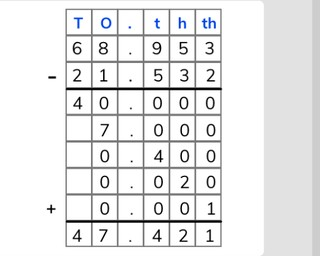Partial differences subtraction with decimal numbers to the thousandths place

# Partial differences subtraction with decimal numbers to the thousandths place

Students learn to use partial differences to subtract decimal numbers to 1,000th8,000 schools use Gynzy92,000 teachers use Gynzy1,600,000 students use Gynzy

## General

Students learn to subtract decimal numbers to the thousandths place using partial differences.

6.NS.B.3

## Relevance

Using partial differences helps you to subtract numbers that are difficult to subtract in one step in your head. You can use it to subtract multiple decimal numbers from each other.

## Introduction

The interactive whiteboard has a number line from 6 to 7 with four arrows. Students must choose which numbers match the arrows using the multiple choice options. You can ask the students to write the letter (of the correct number) on a sheet of paper to hold up in class.

## Development

Explain what the numbers after the decimal point mean and are called. Refresh the names of the tenths, hundredths, and thousandths. Explain to students that when sutracting using partial differences that you write the numbers one below the other. Then you subtract from left to right. Tell students that you write the largest number on the top and the smaller number on the bottom. In this case, you start subtracting at the tens. Subtract these numbers. Then subtract the ones, the tenths, hundredths, and thousandths. It is possible when subtracting ones that your bottom number is greater than your top number. You can choose two options to explain this to the students. Option 1: Tell students that you subtract the two and get a negative number which you mark with a minus sign. Option 2: Tell students to "swap" the numbers in their head (setting the larger number above the smaller number) and mark this swap in the column with a minus sign. When you have written all of these partial sums in the boxes provided, you tell students to follow the signs they've written. So the negative numbers, or numbers with the minus sign (depending on your explanation) are subtracted from the numbers with a plus sign (or without any sign). All together, these numbers total your difference. Show students a problem in which the problem is written in but where they need to write in the partial differences. Ask students to write down the partial differences on a sheet of paper and hold them up so that they can be checked. Next show three problems that students must solve using partial differences where the problems have already been written into the boxes. Then have students complete an entire problem including writing the problems into the boxes. At the end challenge students with a problem which has three numbers.

Check that students are able to subtract decimal numbers to 100 using partial differences by asking the following questions/giving the following tasks:
- Why is it useful to subtract using partial differences?
- Which side do you start when using partial differences?
- Solve the problems: 43.85 - 21.64 = ? and 54.851 - 31.563 = ?

## Guided practice

Students practice subtracting decimal numbers using partial differences by being asked to fill in the partial differences in given problems, by writing in the partial differences and total difference, and by writing in the problem and then solving using partial differences.

## Closing

Discuss with students that it is important to be able to subtract decimals numbers to the thousandths place using partial differences to make it easier to subtract decimal numbers. Check that students know to start solving partial sums from the left to the right. The interactive whiteboard shows a kid who is sharing how much money they have saved in their piggybank, and what they hope to buy. Ask students to use partial differences to solve how much money they'll have left after buying their object.

## Teaching tips

Students who have difficulty with subtracting using partial differences can be supported by refreshing the place value (H,T,O) of numbers and how they belong in a place value chart. Students can also be helped by going through the process of subtracting a larger number from a smaller number. When the number on the bottom is larger than the number on the top it is easiest to explain to students that they need to "swap" the numbers and subtract. But that they must mark that with a minus sign in the boxes where they write their partial difference.

### The online teaching platform for interactive whiteboards and displays in schools

• Save time building lessons

• Manage the classroom more efficiently

• Increase student engagement